Скачать презентацию Chapter 5 Parallel Circuits Topics Covered in Chapter

• Количество слайдов: 23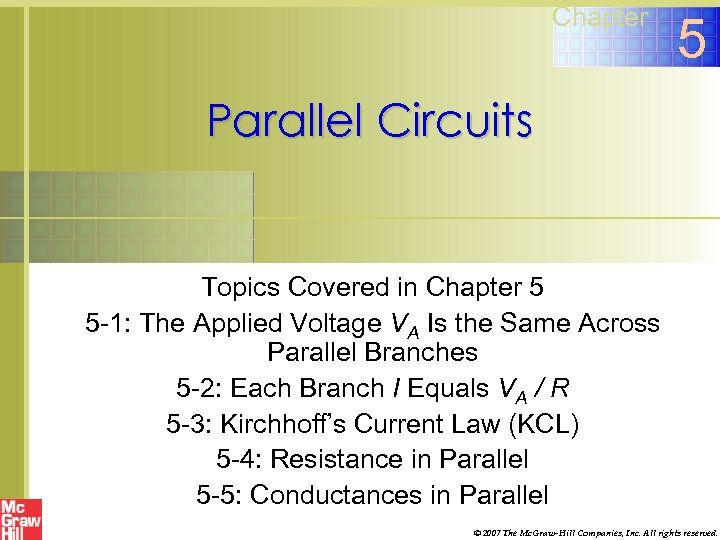Chapter 5 Parallel Circuits Topics Covered in Chapter 5 5 -1: The Applied Voltage VA Is the Same Across Parallel Branches 5 -2: Each Branch I Equals VA / R 5 -3: Kirchhoff’s Current Law (KCL) 5 -4: Resistance in Parallel 5 -5: Conductances in Parallel © 2007 The Mc. Graw-Hill Companies, Inc. All rights reserved.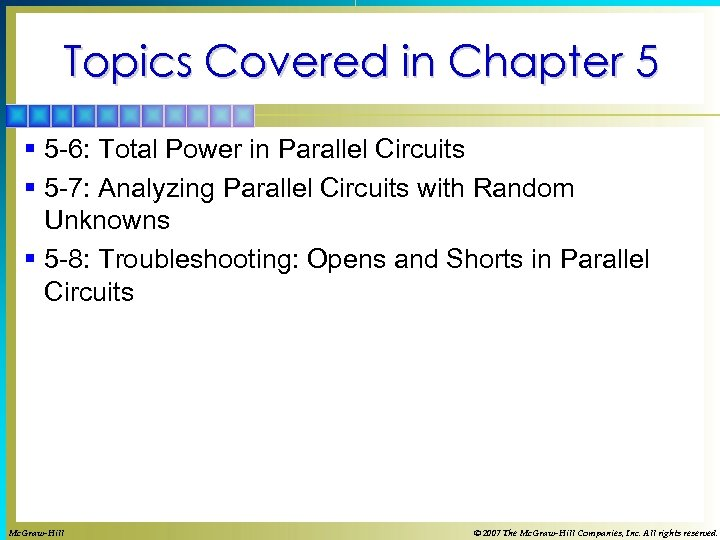Topics Covered in Chapter 5 § 5 -6: Total Power in Parallel Circuits § 5 -7: Analyzing Parallel Circuits with Random Unknowns § 5 -8: Troubleshooting: Opens and Shorts in Parallel Circuits Mc. Graw-Hill © 2007 The Mc. Graw-Hill Companies, Inc. All rights reserved.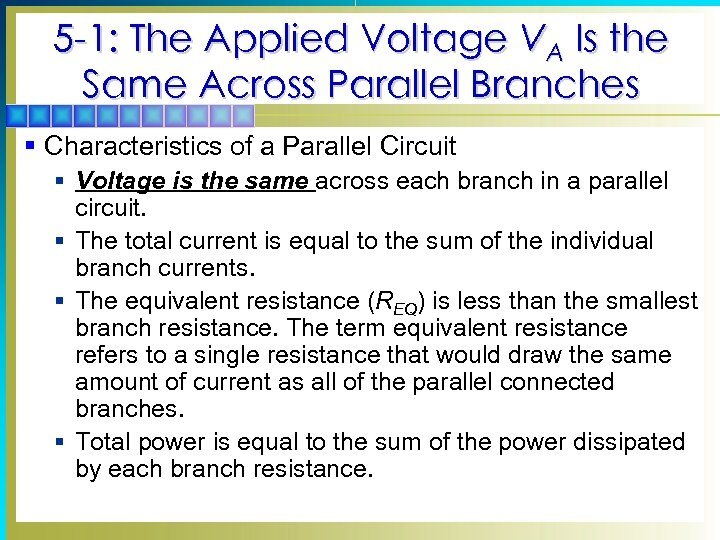5 -1: The Applied Voltage VA Is the Same Across Parallel Branches § Characteristics of a Parallel Circuit § Voltage is the same across each branch in a parallel circuit. § The total current is equal to the sum of the individual branch currents. § The equivalent resistance (REQ) is less than the smallest branch resistance. The term equivalent resistance refers to a single resistance that would draw the same amount of current as all of the parallel connected branches. § Total power is equal to the sum of the power dissipated by each branch resistance.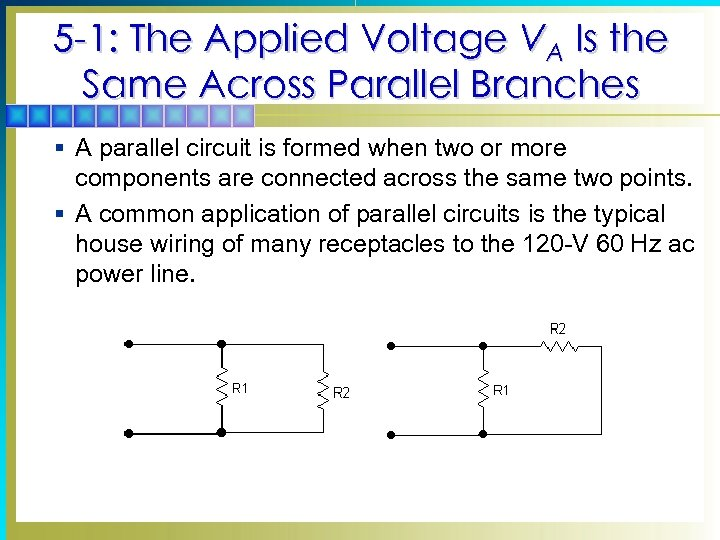5 -1: The Applied Voltage VA Is the Same Across Parallel Branches § A parallel circuit is formed when two or more components are connected across the same two points. § A common application of parallel circuits is the typical house wiring of many receptacles to the 120 -V 60 Hz ac power line.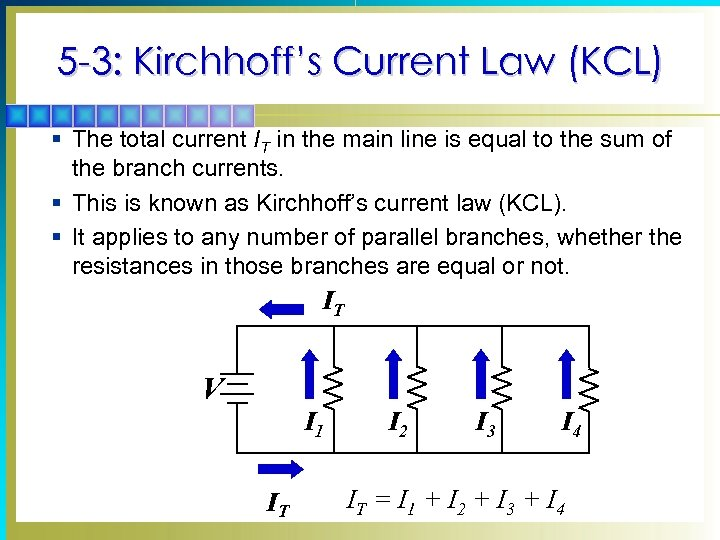5 -3: Kirchhoff’s Current Law (KCL) § The total current IT in the main line is equal to the sum of the branch currents. § This is known as Kirchhoff’s current law (KCL). § It applies to any number of parallel branches, whether the resistances in those branches are equal or not. IT V I 1 IT I 2 I 3 I 4 IT = I 1 + I 2 + I 3 + I 4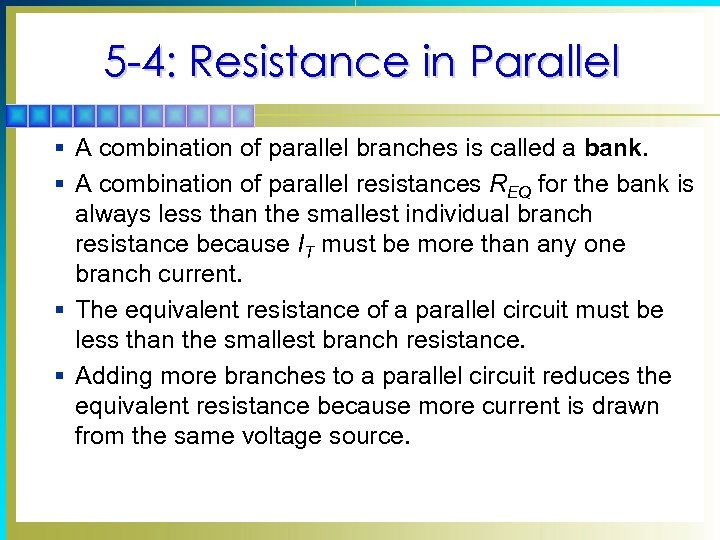5 -4: Resistance in Parallel § A combination of parallel branches is called a bank. § A combination of parallel resistances REQ for the bank is always less than the smallest individual branch resistance because IT must be more than any one branch current. § The equivalent resistance of a parallel circuit must be less than the smallest branch resistance. § Adding more branches to a parallel circuit reduces the equivalent resistance because more current is drawn from the same voltage source.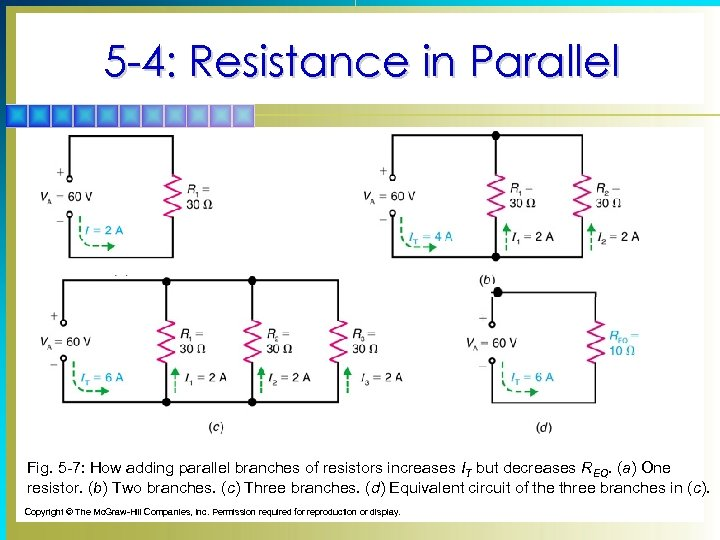5 -4: Resistance in Parallel Fig. 5 -7: How adding parallel branches of resistors increases IT but decreases REQ. (a) One resistor. (b) Two branches. (c) Three branches. (d) Equivalent circuit of the three branches in (c). Copyright © The Mc. Graw-Hill Companies, Inc. Permission required for reproduction or display.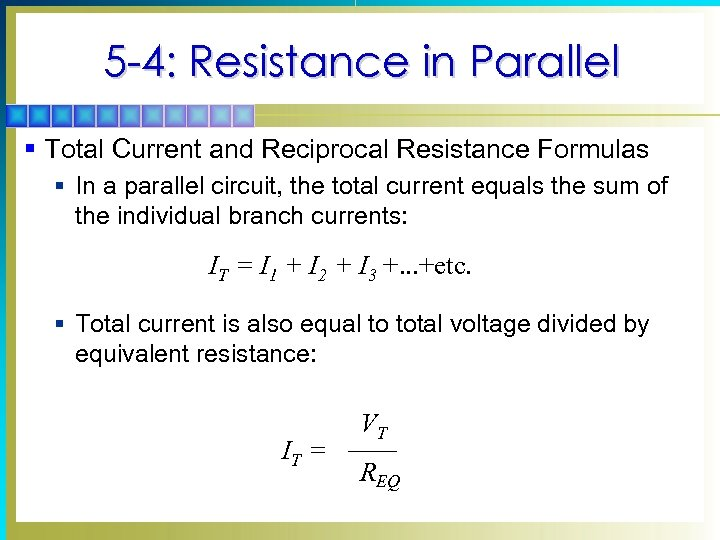5 -4: Resistance in Parallel § Total Current and Reciprocal Resistance Formulas § In a parallel circuit, the total current equals the sum of the individual branch currents: IT = I 1 + I 2 + I 3 +. . . +etc. § Total current is also equal to total voltage divided by equivalent resistance: IT = VT REQ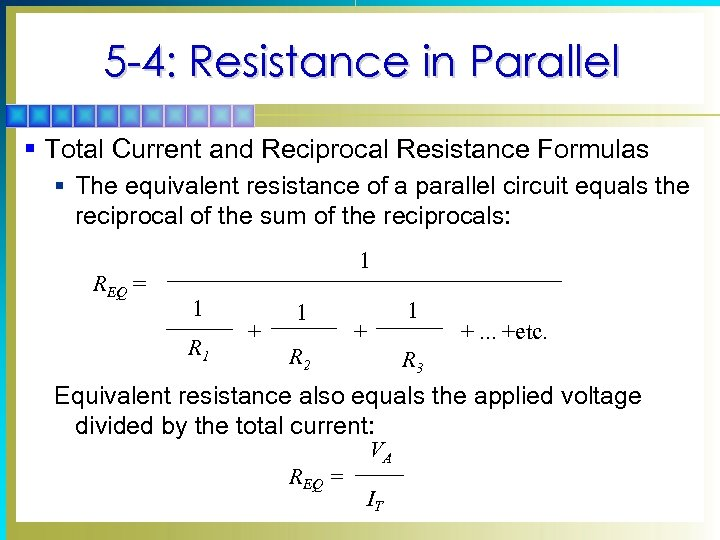5 -4: Resistance in Parallel § Total Current and Reciprocal Resistance Formulas § The equivalent resistance of a parallel circuit equals the reciprocal of the sum of the reciprocals: REQ = 1 1 R 1 + 1 R 2 1 + +. . . +etc. R 3 Equivalent resistance also equals the applied voltage divided by the total current: REQ = VA IT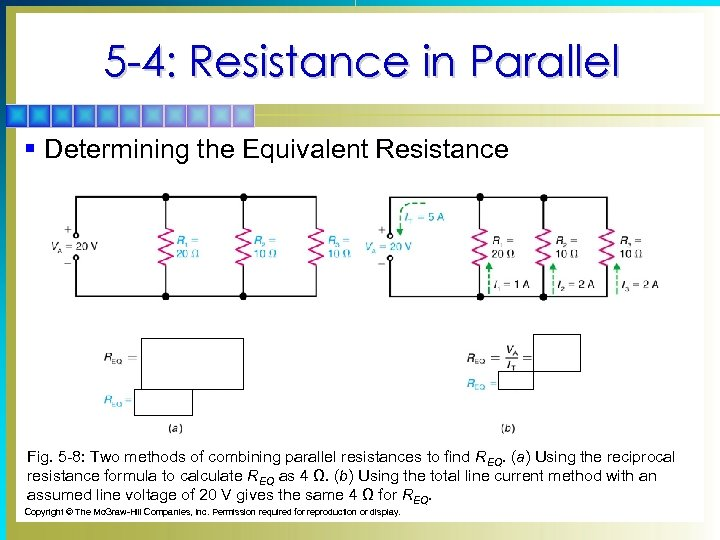5 -4: Resistance in Parallel § Determining the Equivalent Resistance Fig. 5 -8: Two methods of combining parallel resistances to find REQ. (a) Using the reciprocal resistance formula to calculate REQ as 4 Ω. (b) Using the total line current method with an assumed line voltage of 20 V gives the same 4 Ω for REQ. Copyright © The Mc. Graw-Hill Companies, Inc. Permission required for reproduction or display.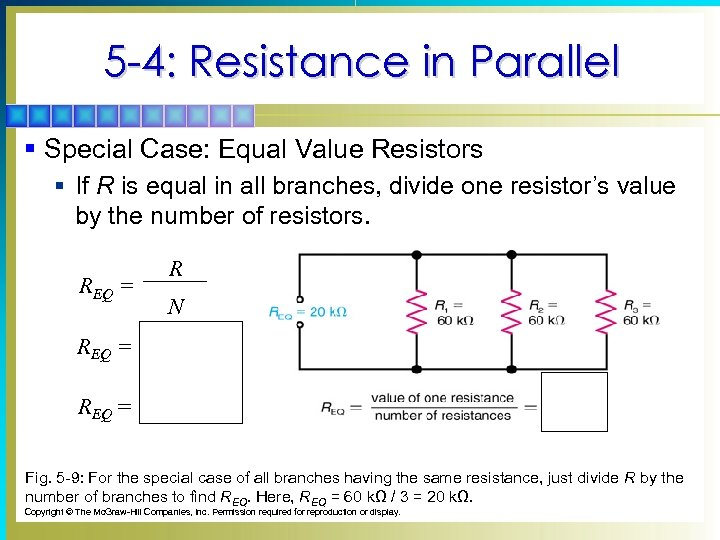5 -4: Resistance in Parallel § Special Case: Equal Value Resistors § If R is equal in all branches, divide one resistor’s value by the number of resistors. REQ = R N 60 kΩ REQ = 3 resistors REQ = 20 kΩ Fig. 5 -9: For the special case of all branches having the same resistance, just divide R by the number of branches to find REQ. Here, REQ = 60 kΩ / 3 = 20 kΩ. Copyright © The Mc. Graw-Hill Companies, Inc. Permission required for reproduction or display.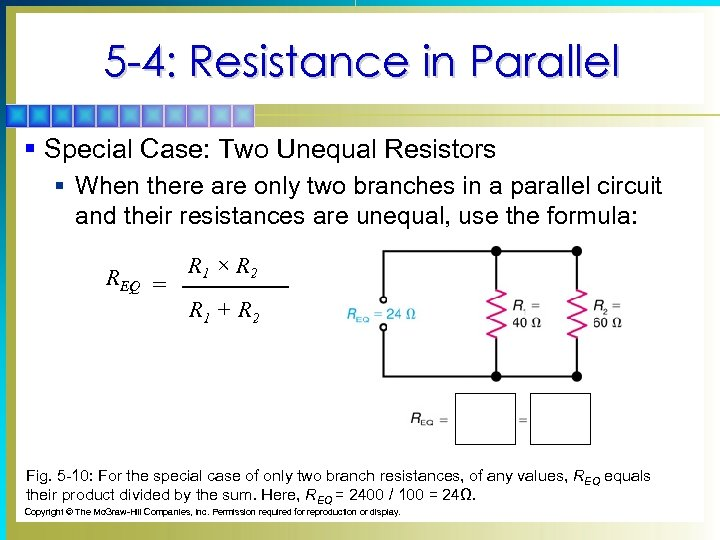5 -4: Resistance in Parallel § Special Case: Two Unequal Resistors § When there are only two branches in a parallel circuit and their resistances are unequal, use the formula: REQ = R 1 × R 2 R 1 + R 2 Fig. 5 -10: For the special case of only two branch resistances, of any values, REQ equals their product divided by the sum. Here, REQ = 2400 / 100 = 24Ω. Copyright © The Mc. Graw-Hill Companies, Inc. Permission required for reproduction or display.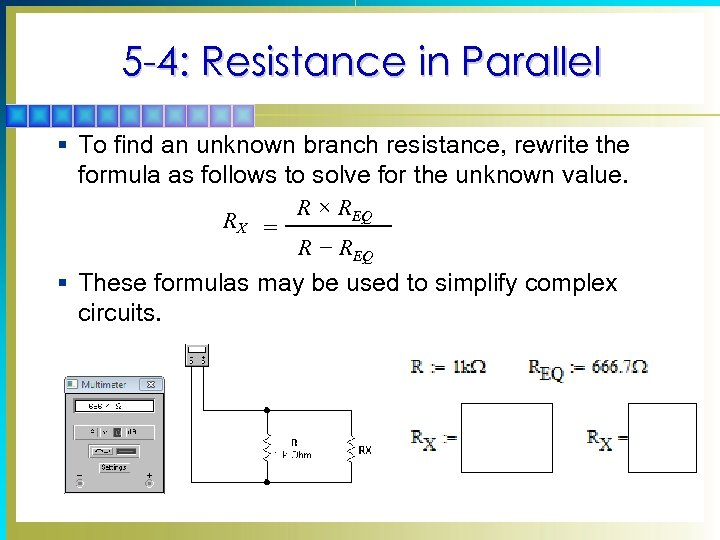5 -4: Resistance in Parallel § To find an unknown branch resistance, rewrite the formula as follows to solve for the unknown value. RX = R × REQ R − REQ § These formulas may be used to simplify complex circuits.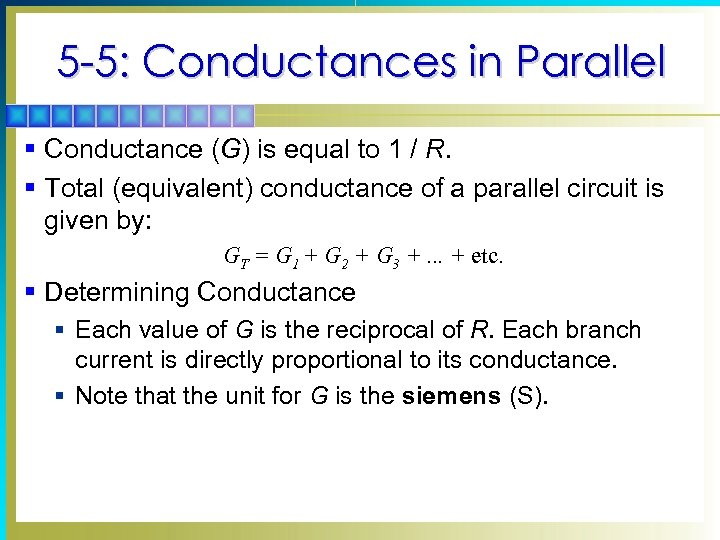5 -5: Conductances in Parallel § Conductance (G) is equal to 1 / R. § Total (equivalent) conductance of a parallel circuit is given by: GT = G 1 + G 2 + G 3 +. . . + etc. § Determining Conductance § Each value of G is the reciprocal of R. Each branch current is directly proportional to its conductance. § Note that the unit for G is the siemens (S).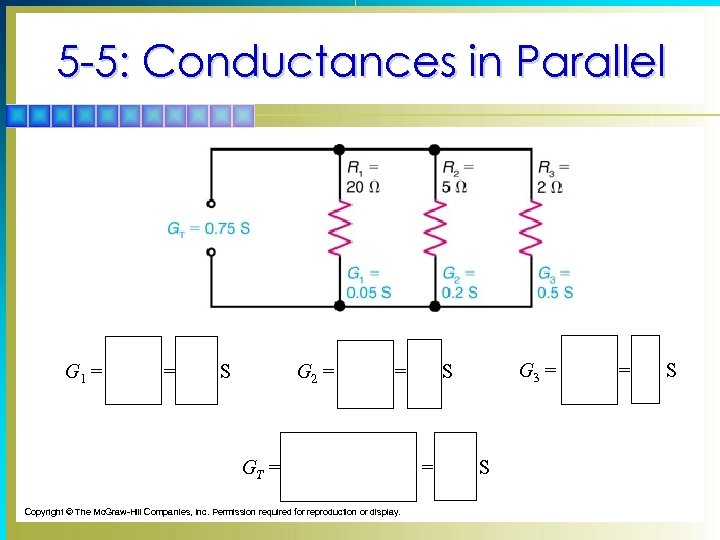5 -5: Conductances in Parallel G 1 = 1 20 W = 0. 05 S G 2 = 1 5 W = 0. 2 S GT = 0. 05 + 0. 2 + 0. 5 = 0. 75 S Copyright © The Mc. Graw-Hill Companies, Inc. Permission required for reproduction or display. G 3 = 1 2 W = 0. 5 S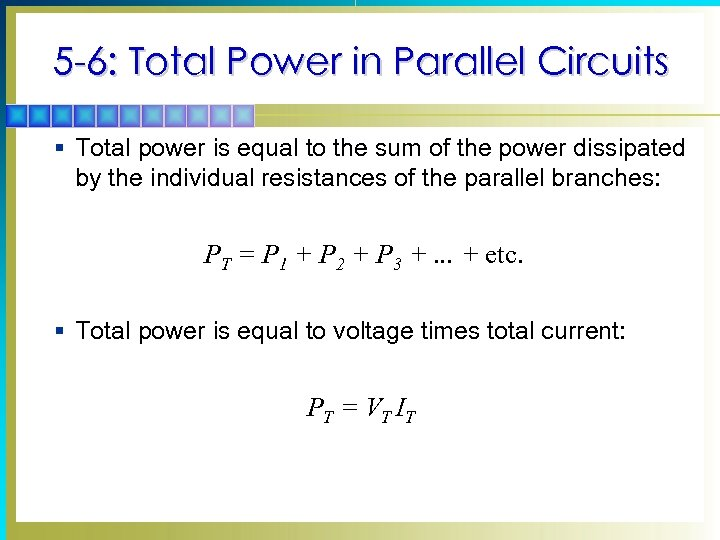5 -6: Total Power in Parallel Circuits § Total power is equal to the sum of the power dissipated by the individual resistances of the parallel branches: PT = P 1 + P 2 + P 3 +. . . + etc. § Total power is equal to voltage times total current: P T = V T IT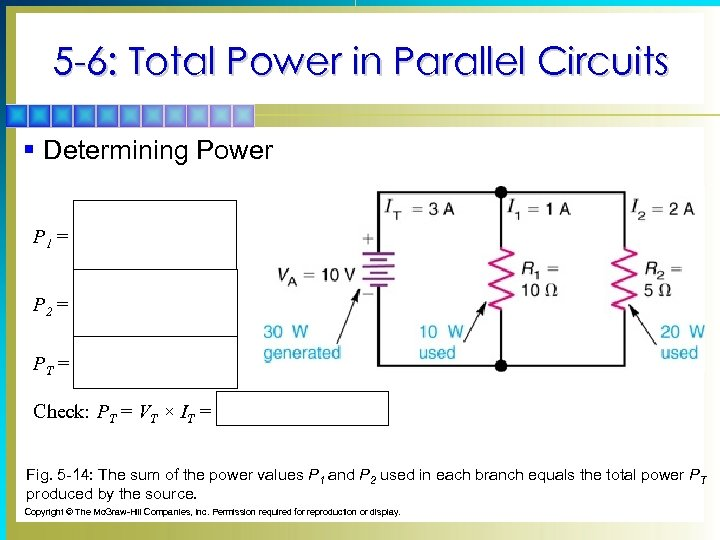5 -6: Total Power in Parallel Circuits § Determining Power P 1 = P 2 = 102 10 W 102 5 W = 10 W = 20 W PT = 10 + 20 = 30 W Check: PT = VT × IT = 10 V × 3 A = 30 W Fig. 5 -14: The sum of the power values P 1 and P 2 used in each branch equals the total power PT produced by the source. Copyright © The Mc. Graw-Hill Companies, Inc. Permission required for reproduction or display.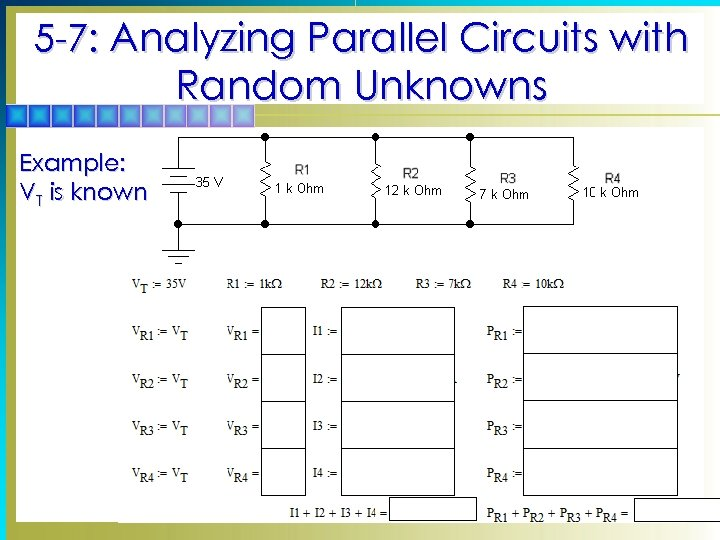5 -7: Analyzing Parallel Circuits with Random Unknowns Example: VT is known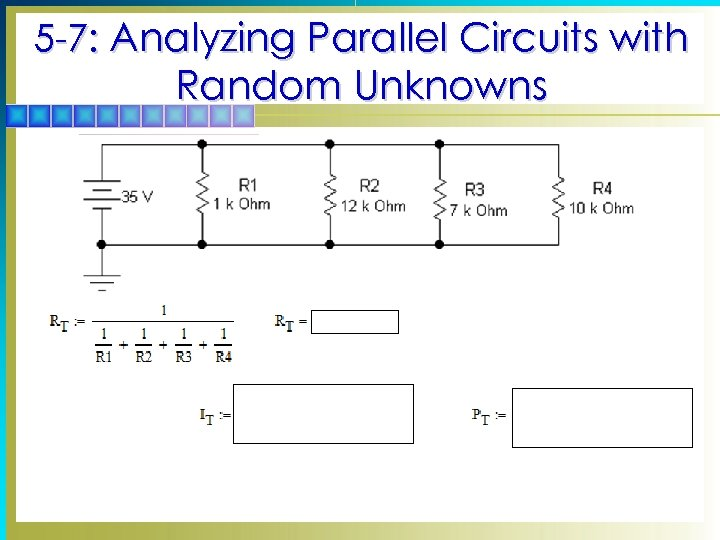5 -7: Analyzing Parallel Circuits with Random Unknowns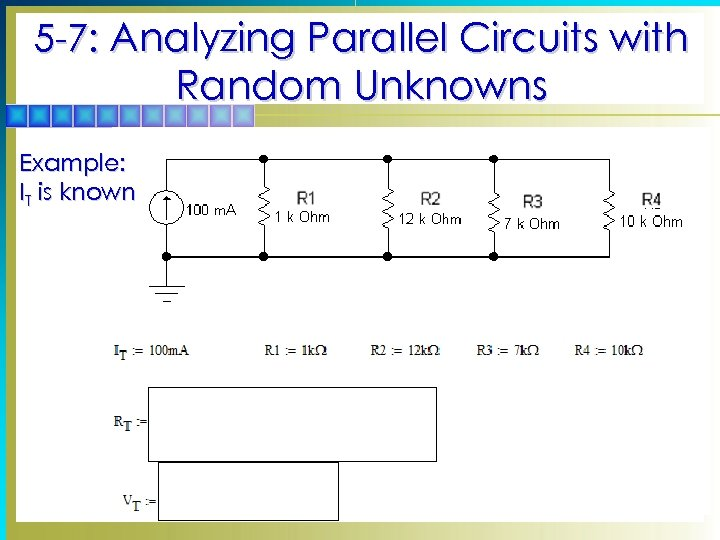5 -7: Analyzing Parallel Circuits with Random Unknowns Example: IT is known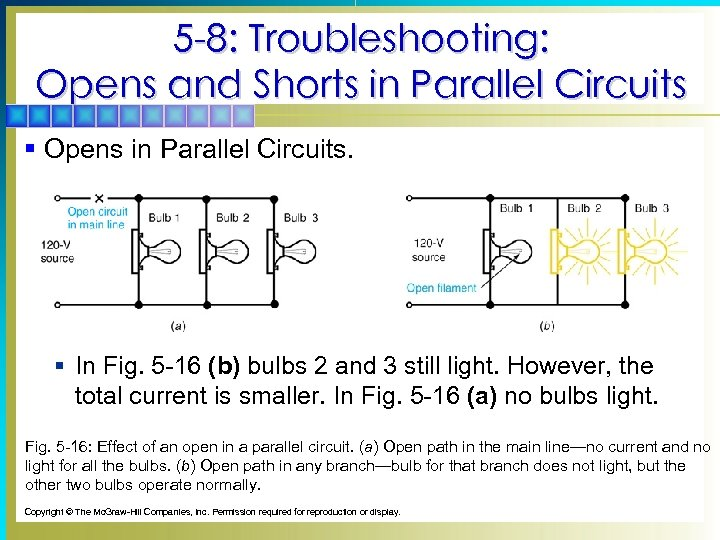5 -8: Troubleshooting: Opens and Shorts in Parallel Circuits § Opens in Parallel Circuits. § In Fig. 5 -16 (b) bulbs 2 and 3 still light. However, the total current is smaller. In Fig. 5 -16 (a) no bulbs light. Fig. 5 -16: Effect of an open in a parallel circuit. (a) Open path in the main line—no current and no light for all the bulbs. (b) Open path in any branch—bulb for that branch does not light, but the other two bulbs operate normally. Copyright © The Mc. Graw-Hill Companies, Inc. Permission required for reproduction or display.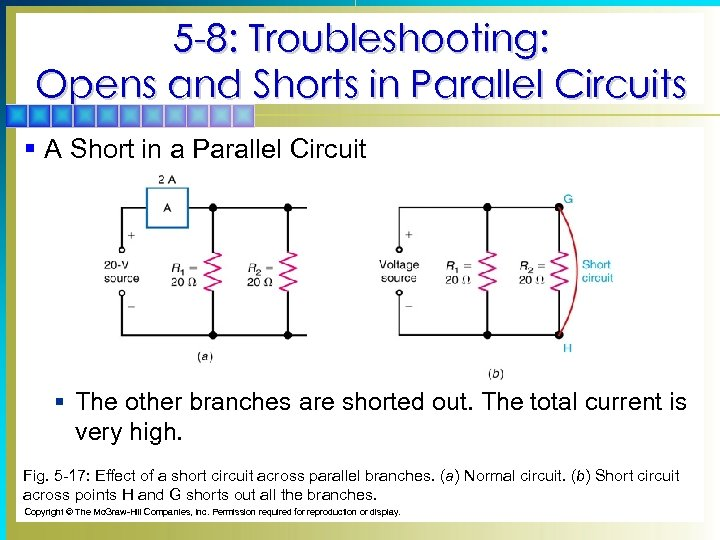5 -8: Troubleshooting: Opens and Shorts in Parallel Circuits § A Short in a Parallel Circuit § The other branches are shorted out. The total current is very high. Fig. 5 -17: Effect of a short circuit across parallel branches. (a) Normal circuit. (b) Short circuit across points H and G shorts out all the branches. Copyright © The Mc. Graw-Hill Companies, Inc. Permission required for reproduction or display.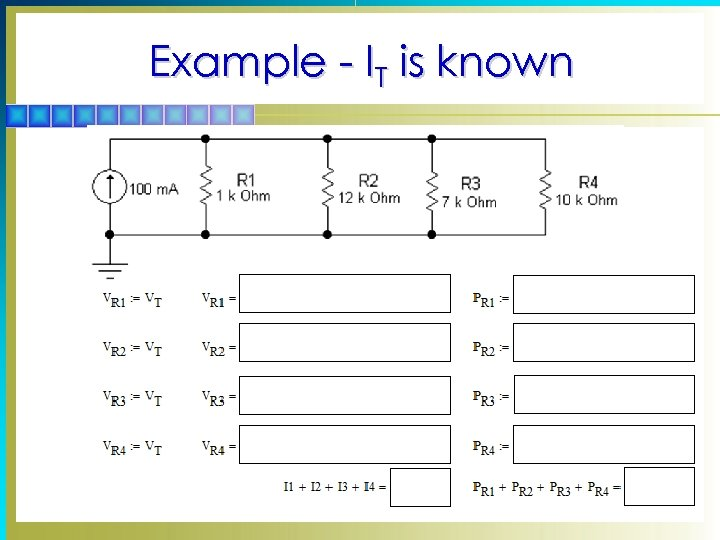Example - IT is known